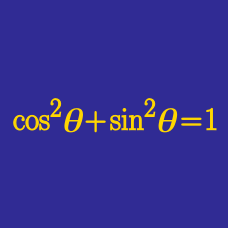Geometry

# Fundamental Trigonometric Identities: Level 3 Challenges

$\sin ^{ 2 }{ 1^{\circ} } +\sin ^{ 2 }{ 2^{\circ} } +\sin ^{ 2 }{ 3^{\circ} } + \ldots \\ +\sin ^{ 2 }{ 88^{\circ} } +\sin ^{ 2 }{ 89^{\circ} } +\sin ^{ 2 }{ 90^{\circ} } = \ ?$

$\large \frac { \sin ^{ 2 }{ \theta } }{ 5 } =\frac { \cos ^{ 2 }{ \theta } }{ 6 }$

If $$\theta$$ is a positive acute angle that satisfies the equation above, find $$\sin { \theta }$$.

Note: Give your answer to 3 decimal places.

$\displaystyle \sum_{k=1}^{50} \Bigg [ \bigg(1 + \tan(k^\circ)+\sec(k^\circ)\bigg)\ \bigg(1+\cot(k^\circ)-\csc(k^\circ) \bigg)\Bigg]=\ ? \$

$\Large \left(\sqrt{2+\sqrt{2}}\right)^{x} + \left(\sqrt{2-\sqrt{2}}\right)^{x} = 2^{x}$

Find the sum of all real $$x$$ that satisfy the equation above.

$\large\frac { 1 }{ \cos ^{ 2 }{ \theta } } +\frac { 1 }{ 1+\sin ^{ 2 }{ \theta } } +\frac { 2 }{ 1+\sin ^{ 4 }{ \theta } } +\frac { 4 }{ 1+\sin ^{ 8 }{ \theta } }$

If $$\large \sin ^{ 16 }{ \theta } = \frac { 1 }{ 5 }$$, what is the value of the expression above?

×

Problem Loading...

Note Loading...

Set Loading...